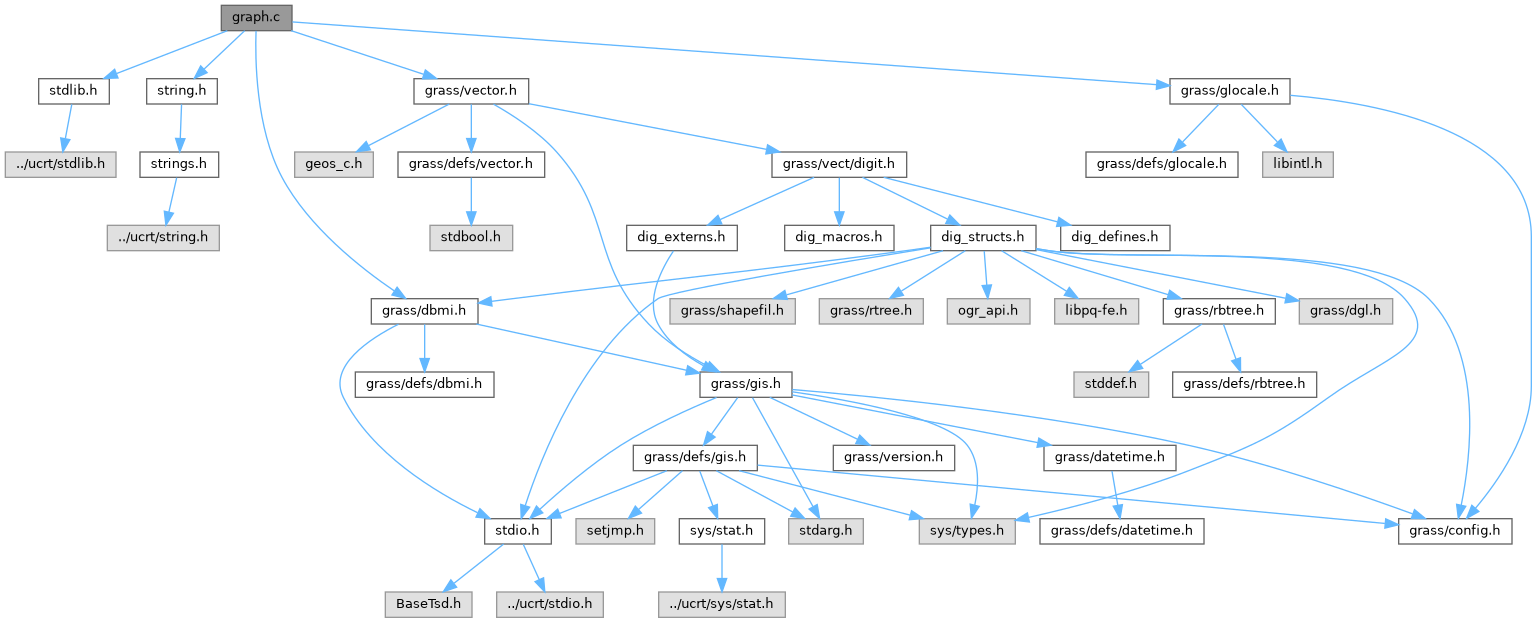GRASS GIS 8 Programmer's Manual  8.2.2dev(2023)-5c00b4e944
vector/Vlib/graph.c File Reference

Vector library - graph manipulation. More...

`#include <stdlib.h>`
`#include <string.h>`
`#include <grass/dbmi.h>`
`#include <grass/vector.h>`
`#include <grass/glocale.h>`
Include dependency graph for vector/Vlib/graph.c:Go to the source code of this file.

## Functions

void Vect_graph_init (dglGraph_s *graph, int nodes_costs)
Initialize graph structure. More...

void Vect_graph_build (dglGraph_s *graph)
Build network graph. More...

void Vect_graph_add_edge (dglGraph_s *graph, int from, int to, double costs, int id)

void Vect_graph_set_node_costs (dglGraph_s *graph, int node, double costs)
Set node costs. More...

int Vect_graph_shortest_path (dglGraph_s *graph, int from, int to, struct ilist *List, double *cost)
Find shortest path. More...

## Detailed Description

Vector library - graph manipulation.

Higher level functions for reading/writing/manipulating vectors.

Todo:
Vect_graph_free ( dglGraph_s *graph )

(C) 2001-2009 by the GRASS Development Team

This program is free software under the GNU General Public License (>=v2). Read the file COPYING that comes with GRASS for details.

Definition in file vector/Vlib/graph.c.

## Function Documentation

 void Vect_graph_add_edge ( dglGraph_s * graph, int from, int to, double costs, int id )

Internal format for edge costs is integer, costs are multiplied before conversion to int by 1000. Costs -1 for infinity i.e. arc or node is closed and cannot be traversed.

Parameters
 graph pointer to graph structure from from node to to node costs costs value id edge id
Returns
void

Definition at line 124 of file vector/Vlib/graph.c.

References _, dglAddEdge(), G_debug(), and G_fatal_error().

## ◆ Vect_graph_build()

 void Vect_graph_build ( dglGraph_s * graph )

Build network graph.

Internal format for edge costs is integer, costs are multiplied before conversion to int by 1000. Costs -1 for infinity i.e. arc or node is closed and cannot be traversed.

Parameters
 graph pointer to graph structure
Returns
void

Definition at line 97 of file vector/Vlib/graph.c.

References _, dglFlatten(), G_debug(), and G_fatal_error().

## ◆ Vect_graph_init()

 void Vect_graph_init ( dglGraph_s * graph, int nodes_costs )

Initialize graph structure.

Parameters
 graph pointer to graph structure nodes_costs use node costs
Returns
void

Definition at line 71 of file vector/Vlib/graph.c.

References dglInitialize(), and G_debug().

## ◆ Vect_graph_set_node_costs()

 void Vect_graph_set_node_costs ( dglGraph_s * graph, int node, double costs )

Set node costs.

Internal format for edge costs is integer, costs are multiplied before conversion to int by 1000. Costs -1 for infinity i.e. arc or node is closed and cannot be traversed.

Parameters
 graph pointer to graph structure node node id costs costs value
Returns
void

Definition at line 154 of file vector/Vlib/graph.c.

References dglGetNode(), dglNodeSet_Attr(), and G_debug().

## ◆ Vect_graph_shortest_path()

 int Vect_graph_shortest_path ( dglGraph_s * graph, int from, int to, struct ilist * List, double * cost )

Find shortest path.

Costs for 'from' and 'to' nodes are not considered (SP found even if 'from' or 'to' are 'closed' (costs = -1) and costs of these nodes are not added to SP costs result.

Parameters
 graph pointer to graph structure from from node to to node List list of line ids cost costs value
Returns
number of segments
0 is correct for from = to, or List == NULL ), ? sum of costs is better return value,
-1 destination unreachable

Definition at line 184 of file vector/Vlib/graph.c.